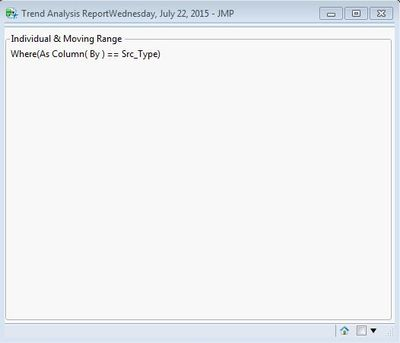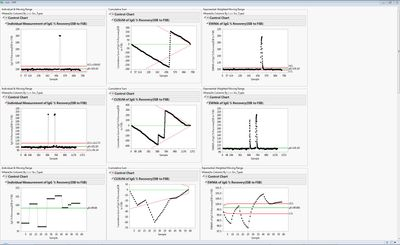Highlighted

## Empty Control Chart Windows..........Weird Situation.

Hello,

I'm trying to plot three control charts(IR,EWMA & CUSUM) but for some weird reason the chart windows are showing blank space. When tried using JMP interface it's plotting the charts perfectly.

One interesting fact is that same chart is getting populated when performing 3rd step mentioned below in case of first run to generate limits but not when actually required with limits even the limits currently being applied is calculated from the same table.

This is how my code flow works:

-> Get input files

->Join them to create main table

->Calculate Limits and store in another table for all process parameters.(For first run limits will be get calculated from main table created in previous step)

->In plot function Loop on Parameters and get limits for them and call display function

->Plot the charts using main table values while applying appropriate limit values.

Here is the code for last two steps:

display=Function({Process,Label,By,Src_Type,lmt1,lmt2,lmt3},

r1=New Window("Trend Analysis Report" ||Long Date(Today()),

H List Box(

Panel Box("Individual & Moving Range",Current Data Table(dt);//dt is main table from Step 2.

cc=Control Chart(

Sample Label(As Name(Label)),

Group Size(1),KSigma(3),

As Column(Process),Individual Measurement(LCL(lmt1),AVG(lmt1),UCL(lmt1))

),

Where(As Column(By)==Src_Type)

);

),//Excluded other chart types to cut the space short!!

);

);

);

plot=Function({lmts,pmt_path},

param_dt=Open(pmt_path,"invisible");

param_summ=param_dt<<Summary(Invisible,

Group(:Group By,:Process,:Sample Label,:By))<<Show Window(0);

For(q=12,q<=NRow(param_summ),q++,Current Data Table(param_summ);

col2=:Process[q];col3=:Sample Label[q];col4=:By[q];

Current Data Table(lmts);

clms=lmts<<Get Column Names(String);

Remove From(clms,1,3);

For(t=1,t<=N Items(clms),t++,Current Data Table(lmts);

indv_lmts=As Column(clms[t])[lmts<<Get Rows Where(:Parameter==col2 & :Chart Type=="Individual")];

cusum_lmts=As Column(clms[t])[lmts<<Get Rows Where(:Parameter==col2 & :Chart Type=="CUSUM")];

ewma_lmts=As Column(clms[t])[lmts<<Get Rows Where(:Parameter==col2 & :Chart Type=="EWMA")];

//Current Data Table(dt);

//dt1=dt<<get rows where(as column(dt,col4)==clms);

//if(nrows(dt1)>0,dt<<select where(column(dt,col4)!=clms)<<exclude<<clear select;);

display(col2,col3,col4,clms[t],indv_lmts,cusum_lmts,ewma_lmts);

//dt<<clear row states;

);

);

);

Any help on where I'm missing the trick would be very helpful. I tries quite a lot of things but in vain.  #Comment please if I created any kind of confusion.

Thanks!!**Updated: Limit Values used:

LCL = 93.6194355860458;AVG = 101.61918084006;UCL = 109.618926094074;

LCL = 91.1389663749264;AVG = 101.933916247341;UCL = 112.728866119756;

LCL = 95.791125521153;AVG = 99.6808876541094;UCL = 103.570649787066;

LCL = 96.1221889831269;AVG = 99.2913942704352;UCL = 102.460599557744;

LCL = .;AVG = 101.63975868081;UCL = .;

LCL = 96.4170696517472;AVG = 99.4247943691138;UCL = 102.43251908648;

LCL = 85.6694623331952;AVG = 102.356837670718;UCL = 119.044213008241;

LCL = 85.2093412100746;AVG = 105.163584386677;UCL = 125.117827563278;//For this log showing "Control limits out of order: must be LCL<=Avg<=UCL"

2 REPLIES 2
Highlighted

## Re: Empty Control Chart Windows..........Weird Situation.

Seems like there is some issue when trying to get individual window result but when I tried to append all of the loops in one final window result do show up. If anyone can help on this or I'm going some wrong way.

Updated Code:

V=VList Box("All");

display=Function({Process,Label,By,Src_Type,lmt1,lmt2,lmt3},

r1=New Window("Trend Analysis Report" ||Long Date(Today()),

H List Box(

Panel Box("Individual & Moving Range",Current Data Table(dt);//dt is main table from Step 2.

cc=Control Chart(

Sample Label(As Name(Label)),

Group Size(1),KSigma(3),

As Column(Process),Individual Measurement(LCL(lmt1),AVG(lmt1),UCL(lmt1))

),

Where(As Column(By)==Src_Type)

);

),//Excluded other chart types to cut the space short!!

);

);V<<Append(r1);r1<<CloseWindow;

);

plot=Function({lmts,pmt_path},

param_dt=Open(pmt_path,"invisible");

param_summ=param_dt<<Summary(Invisible,

Group(:Group By,:Process,:Sample Label,:By))<<Show Window(0);

For(q=12,q<=NRow(param_summ),q++,Current Data Table(param_summ);

col2=:Process[q];col3=:Sample Label[q];col4=:By[q];

Current Data Table(lmts);

clms=lmts<<Get Column Names(String);

Remove From(clms,1,3);

For(t=1,t<=N Items(clms),t++,Current Data Table(lmts);

indv_lmts=As Column(clms[t])[lmts<<Get Rows Where(:Parameter==col2 & :Chart Type=="Individual")];

cusum_lmts=As Column(clms[t])[lmts<<Get Rows Where(:Parameter==col2 & :Chart Type=="CUSUM")];

ewma_lmts=As Column(clms[t])[lmts<<Get Rows Where(:Parameter==col2 & :Chart Type=="EWMA")];

//Current Data Table(dt);

//dt1=dt<<get rows where(as column(dt,col4)==clms);

//if(nrows(dt1)>0,dt<<select where(column(dt,col4)!=clms)<<exclude<<clear select;);

display(col2,col3,col4,clms[t],indv_lmts,cusum_lmts,ewma_lmts);

//dt<<clear row states;

);

);

);

v=New Window("test",V);The new issue I have is to rather than creating different Panel boxes each time put everything under one and get rid of this "Where(....)" thing from display.

Highlighted

## Re: Empty Control Chart Windows..........Weird Situation.

Just Noticed my Sample Label column is not being included in charts which is a Date column.  Is there anything causing problem to this which I'm not aware of??

Article Labels

There are no labels assigned to this post.